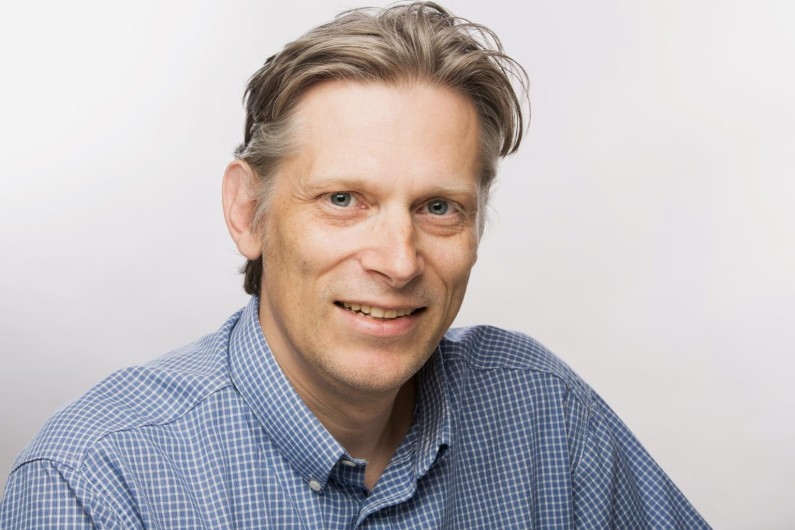• Home
• News
• Joris van der Hoeven awarded for his work on the complexity of multiplication

# Joris van der Hoeven awarded for his work on the complexity of multiplication

The N.G. de Bruijn Medal 2022 has been awarded to Joris van der Hoeven, researcher at the Computer Science Laboratory. The prize was awarded during the Netherlands Mathematical Congress for his research on the complexity of multiplication with David Harvey from the University of New South Wales.20 Apr. 2022
Research, Mathématiques, LIX

Multiplication may be an elementary operation, but it still raises questions for specialists. One in particular is how complex it is, i.e. how much resource is needed to complete the operation in terms of number of steps or computation time? If computers were using the method we learned in school to perform multiplication, it would take them about 30 years to multiply two billion-digit numbers. But it only takes them a few seconds because they use a much more efficient algorithm. Could it be even better?

Joris van der Hoeven, CNRS research director at the Computer Science Laboratory (LIX*), has been working on this problem together with mathematician David Harvey from the University of New South Wales. In 2019, they announced a major result published in the journal Annals of Mathematics: the most efficient multiplication algorithm to date. This result earned Joris van der Hoeven the N.G. de Bruijn Medal. "The prize is intended for researchers of Dutch nationality or working in the Netherlands, but I would obviously like to share it with David Harvey," explains the researcher.

Complexity is a core concept

Estimating algorithm complexity is an important problem for specialists at the interface between mathematics and computer science, not only to compute faster, but also to deepen the mathematical understanding of the problem. Moreover, multiplication is a basic building block for many other algorithms.

If we multiply two n-digit numbers with the algorithm we learned at school, the complexity is proportional to n². But since the 1960s, increasingly efficient algorithms have been discovered. Their general principle is to try to replace as much as possible elementary multiplication by less complex additions. Thus, the algorithm of Schönhage and Strassen, proposed in 1971, had a complexity of the order of n x log n x log (log n), where log stands for the logarithm function.

"But Schönhage and Strassen conjectured that the complexity of the multiplication could be reduced to n x log n, but did not provide a way to do this," explains Joris van der Hoeven, who started working on this problem with David Harvey in 2014. They pushed further a tool already used by the previous methods known as fast Fourier transform. It allows, roughly speaking, to reduce multiplication on n-digit numbers to multiplication on log n-digit numbers. "We have succeeded several times in reducing the complexity, without reaching the n x log n limit. We finally achieved this, to our great surprise," recalls the LIX researcher.

This algorithm is now the fastest known for multiplying, at least in theory. The practical implementation is another story, as it is intimately dependent on the architecture of computer processors. Today's chips optimise multiplication operations much more than additions. Finally, even in theory, the existence of an algorithm with even lower complexity remains open. "Personally, I don't think so," says Joris van der Hoeven, "but we don't have the proof yet".

*LIX: a joint research unit CNRS, École Polytechnique - Institut Polytechnique de Paris